Contents
Error in AMS-SoptS

## Ohtsuka Lab.

The virtual laboratory is the workplace of my independent research after retirement created by my tool MaKR which is recreated with mathematical expressions MathJax . The main topics are more details and additional pictures of AMS-SoptS

AMS-SoptS is the paper Finite element analysis and shape optimization of singular points in boundary value problems for partial differential equations
published in the journal Sugaku Expositions from American Mathematical Society This article originally appeared in Japanese in Sugaku 70 3 (2018), 255--274. However, the symbols have been improved and the results have been added in relation to fracture mechanics and recent results.

## What's SoptS

The theme is named SoptS (Shape Optimisation of Singular Points in BVPs) to study fracture phenomena and (boundary) shape optimisation in terms of singularity of solutions of boundary value problem for partial differential equations (BVPs). SoptS is closely related to fracture mechanics and shape optimisation problems. The main tool in SoptS, the GJ-integral, is a natural extension of the well-known J-integral in fracture mechanics.
(MaKR = Mathematical Knowledge Repository, making since around 2000.)

## Topics

• Mathematical research based on the concept of fracture mechanics starting from graduate school (1980), that is a really difficult problem and has not been solved yet.
• I proposed a gneralization (GJ-integral) of J-integral to express 3-dimensional fracture phenomena in 1981
• Systematic study of shape sensitivity analysis such as Hadamard's variational formula using GJ-integral.
• The method using GJ-integral has features such as being applicable even if the boundary value problem has singular points and also extracting local features

## Fracture

Let $\Omega$ be a bounded domain with Lipschitz boundary in $\mathbb{R}^d, d=2,3$, and $\Sigma \subset \Omega$ be a surface with the edge. The elasticity is defined on the domain $\Omega _{\Sigma }:=\Omega \setminus \Sigma$ with stress free $\sigma _{ij}^{±}(u)\nu _j=0$ on $\Sigma$,where $u$ is the displacement permitting the jump across the crack , $\sigma _{ij}^{±}(u)$ the stress on the both($±$) side of $\Sigma$, and $\nu =(\nu _j)_{j=1,…,d}$ is the unit normal vector on $\Sigma$ directed from the plus side toward the minus. Notice that $u^+(x)=u^-(x)$ on $x\in \partial \Sigma$.
Examining the singularity at the edge of the crack in an elastic body with a crack is called crack problem.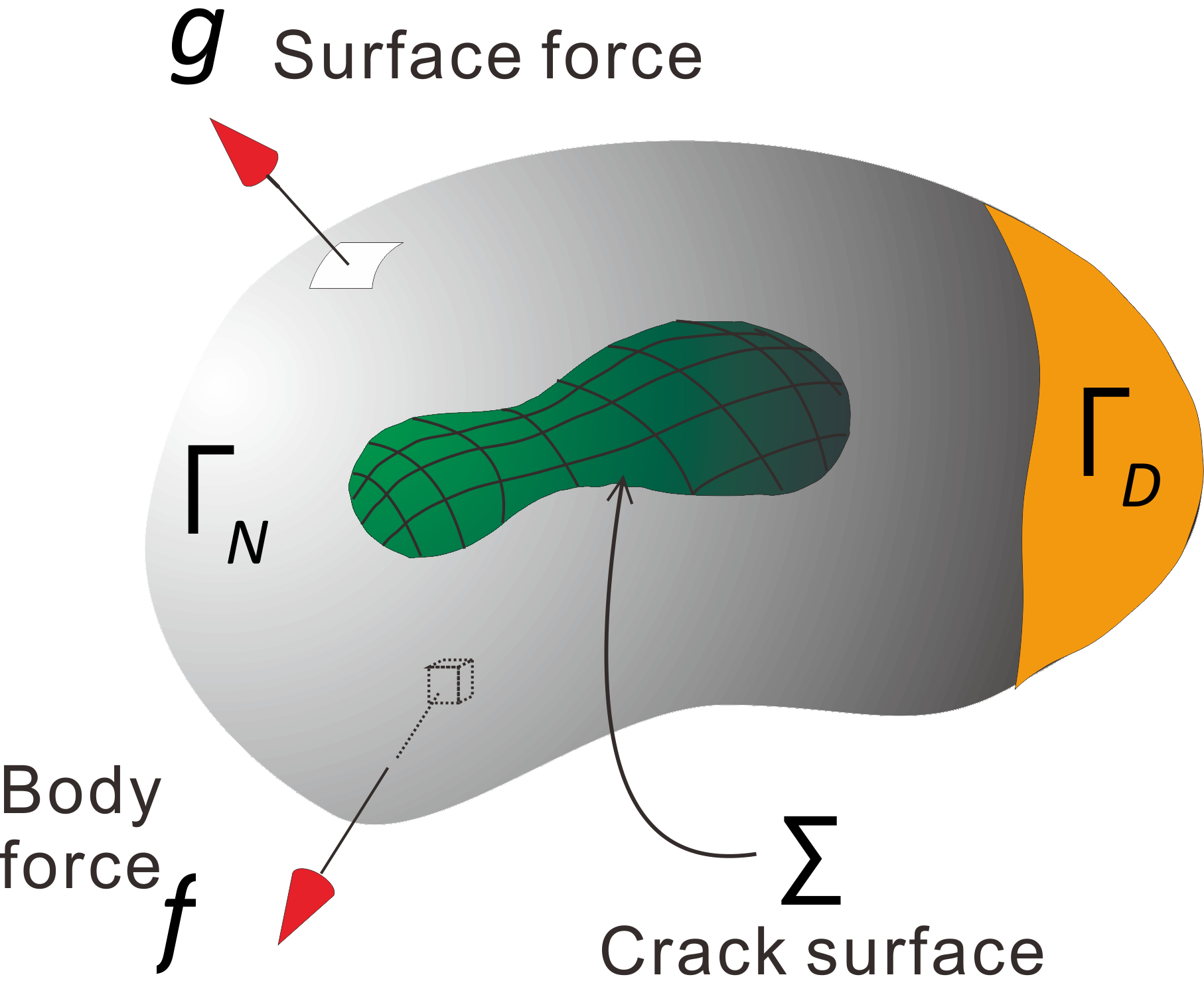Fracture problem is to simulate crack progresses, that is the initiation and propagation of failure, etc. Huge mathematical model describing fracture phenomena has been created, but there is no one to handle phenomena by systematically expressing, For now, part of fracture phenomenon can be analyzed mathematically based on energy balance theory of Griffith.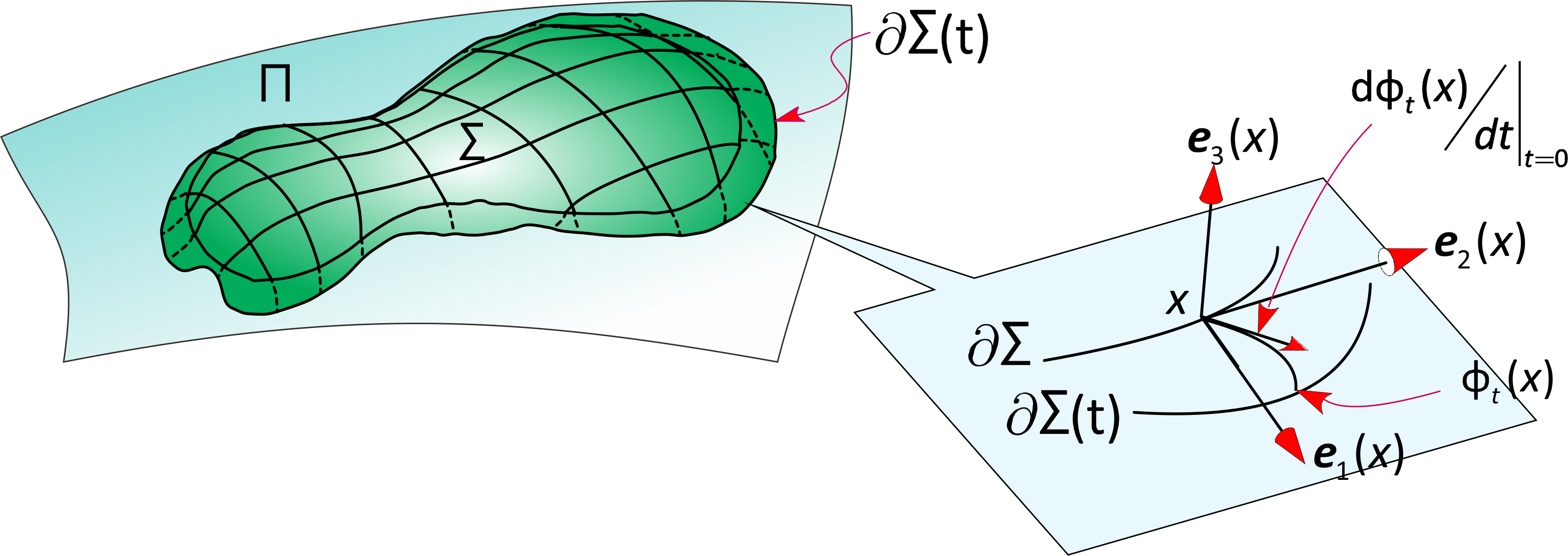More..

## Shape Optimization of boundary

Let $\Omega$ be a bounded domain with Lipschitz boundary in $\mathbb{R}^d, d=2,3$ and $u(\Omega )$ a minimizer of an energy functional $\mathcal{E}$ over a subset $V(\Omega )$ in the function space $W^{1,p}(\Omega ;\mathbb{R}^m),m\ge 1$, Consider a cost functional $F(u(\Omega ))$. The shape optimization of boundary is a problem of finding a method of deforming a given set $\Omega$ so as to lower the cost function, and optimized shape $\Omega ^O$ is given by $$F(u(\Omega ^O)) \lt F(u(\Omega ))$$

### Energy Optimization in Dirichlet boundary conditions

A famous example is the Poisson's equation $-\Delta u=f$ with Dirichlet boundary conditions; $u=0$ on $\partial \Omega$. The minimizer $(u^{OD},\Omega ^{OD})$ of the energy $\mathcal{E}(u)=\int _{\Omega }\{|\nabla u|^2/2-fu\} dx$, that is, $$F(u(\Omega ^{OD}))=\min _{\Omega }\{F(u(\Omega )):|\Omega |=constant\}$$ satisfy the condition $\partial u^{OD}/\partial n=\textrm{constant}$ on $\partial \Omega ^{OD}$. Here $F(u(\Omega ))=\mathcal{E}(u)$. The figure just below is the numerical example when $f=1$.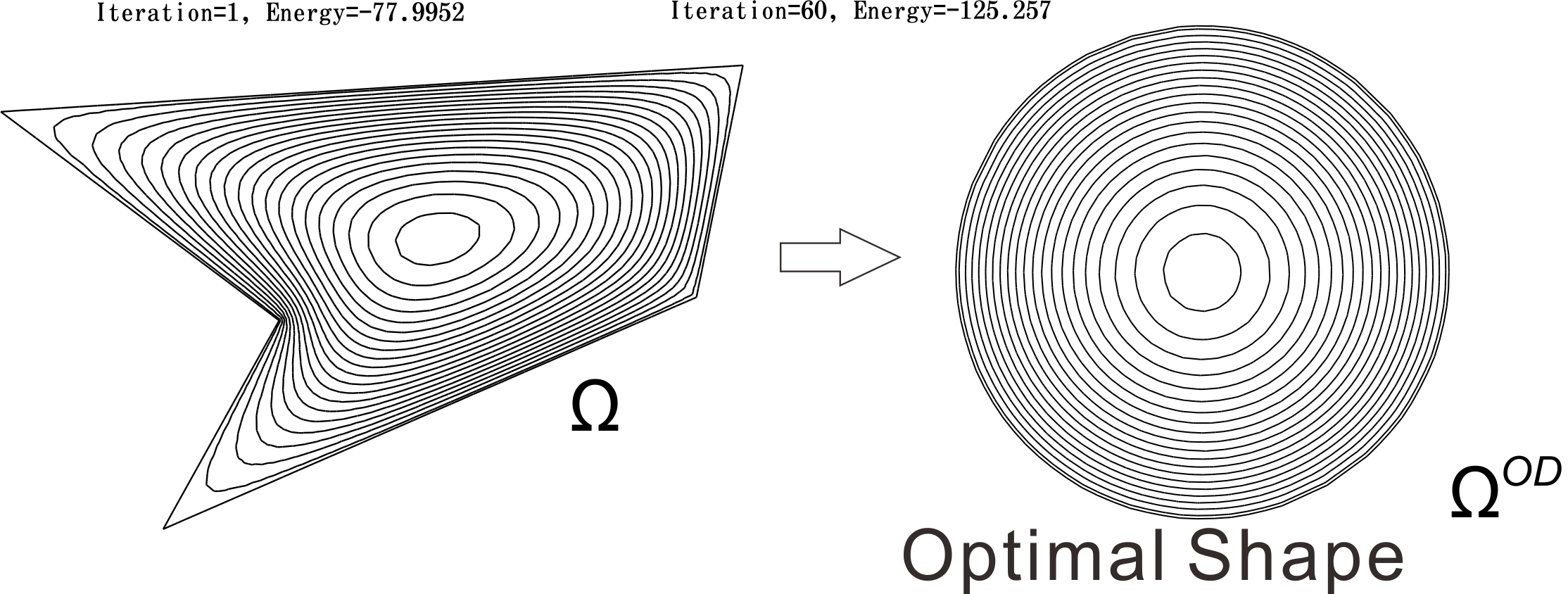Optimal design $\Omega ^{OD}$ obtained by iterative calculation from the polygon on the left

Just because the disk $\Omega ^{OD}$ is energy stable, not all shapes can be made into a disk. It is more practical to think about how to deform the shape to make it more energy stable. We say Shape Optimization Problem to find the method how to deform the shape $\Omega$ to make more stable shape $\Omega ^{O}$. We call $\Omega ^O$ optimized shape, and $\Omega ^{OD}$ (if exists) is called optimal shape (right hand side of the figure just above).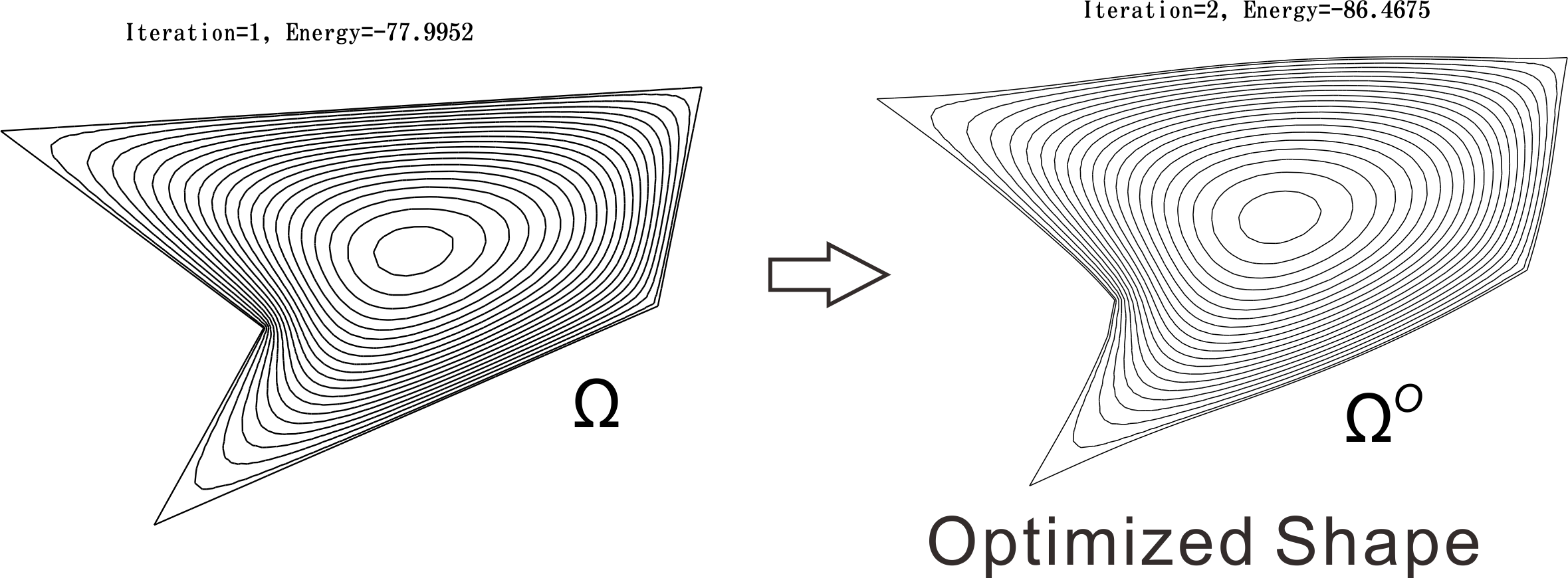An optimized shape of a polygonal domain

### Optimization in Mixed boundary condition

Select the unit disk $\Omega =\{(x_1,x_2):x_1^2+x_2^2 \lt 1\}$ as the initial, $u=0$ on $\Gamma _D=\partial \Omega \cap \{x_2 \gt 0\}$ and put $\partial u/\partial n=0$ on $\Gamma _N=\partial \Omega \cap \{x_2 \lt 0\}$ as shown in the figure just below. The right figure is an optimized shape $\Omega ^O$ of $\Omega$. The solution $u$ has high singularity at $\gamma _M^i,i=1,2$, that is, $u\notin H^{3/2}$ and $u\in H^{3/2-\epsilon }$ near $\gamma _M^i,i=1,2$ for all $\epsilon \gt 0$.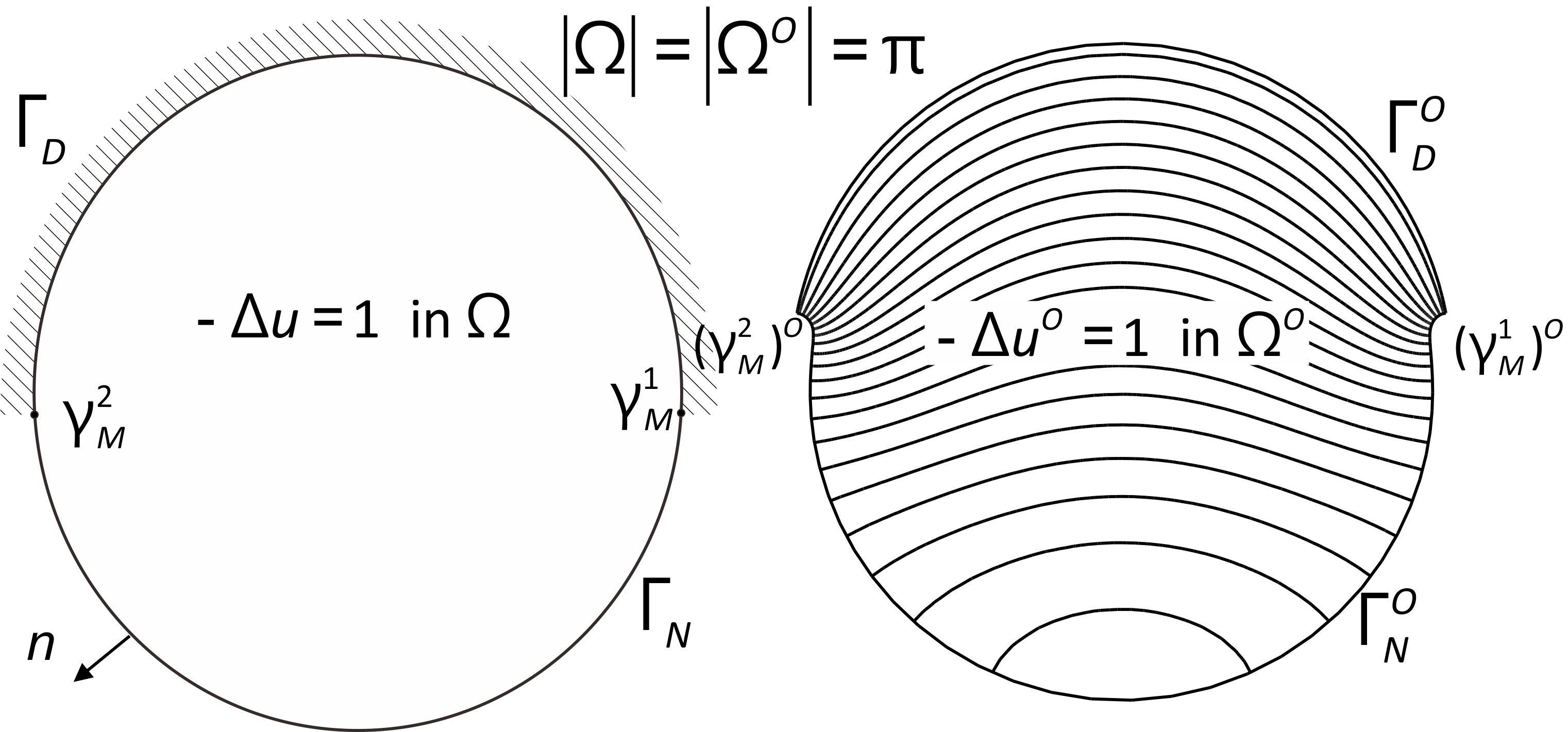Optimized shape in Poisson Eq. with mixed boundary condition
As a feature, the angle at $(\gamma _M^i)^O,i=1,2$ becomes smaller than $\pi$, then $u\in H^{3/2}$ near $(\gamma _M^i)^O$. Second feature is that $|\Gamma _D^O| \lt |\Gamma _D|, |\Gamma _N^O| \gt |\Gamma _D|$ where $|\Gamma |$ denotes the length of the curve $\Gamma$. A series of optimizations as shown in the figure below can be obtained.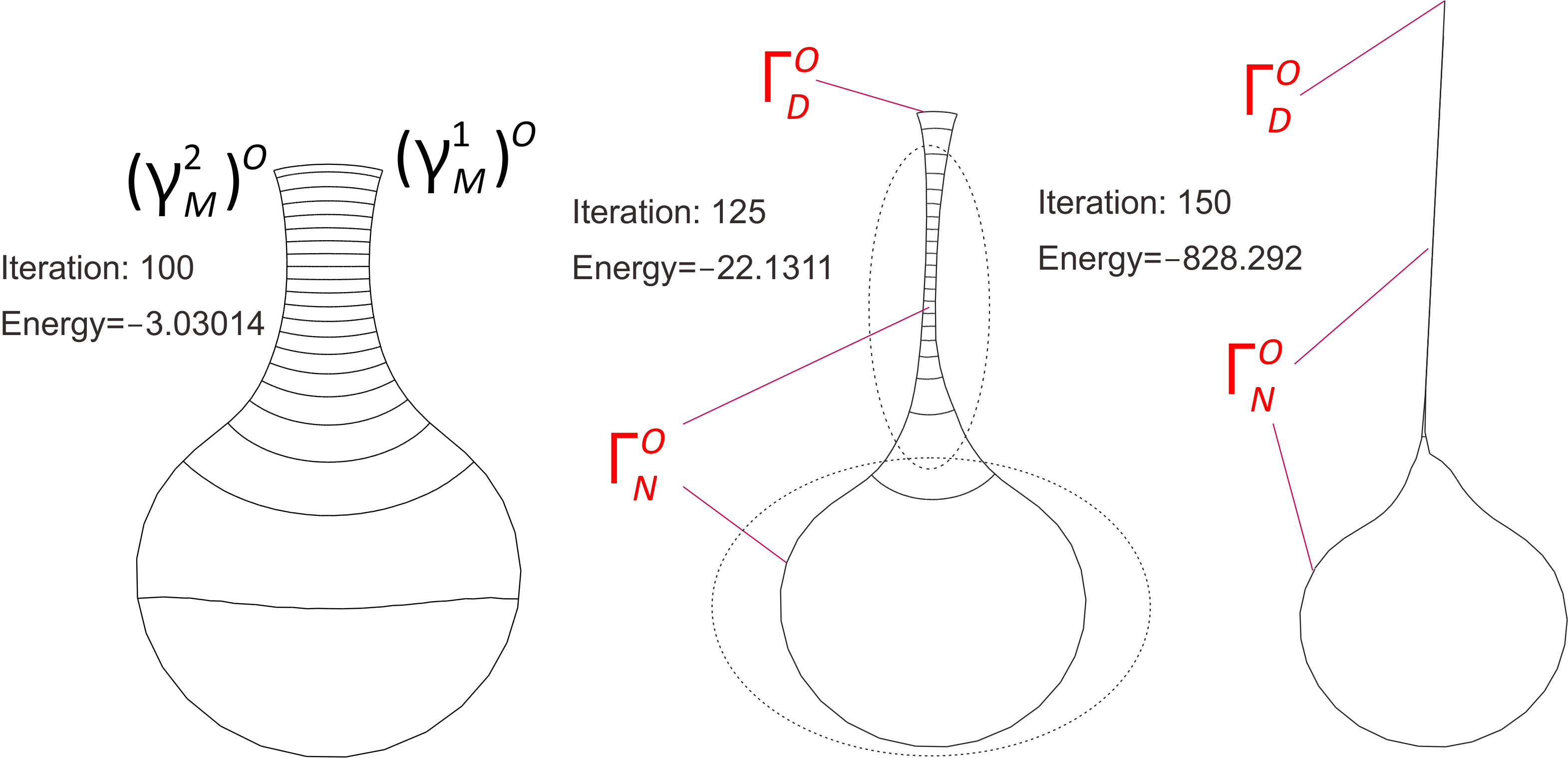A series of optimizations can be obtained.
More..
$\Gamma _D^O$ become small like a dot, and $\Gamma _N^O$ has a shape in which a thread is attached to a disk and connected to $\Gamma _D^O$.
Notice that optimized shapes are determined by $f$ in $-\Delta u=f$. For example $f(x_1,x_2)=\sin (2\pi x_1)$, we get a series of optimized shapes as shown below..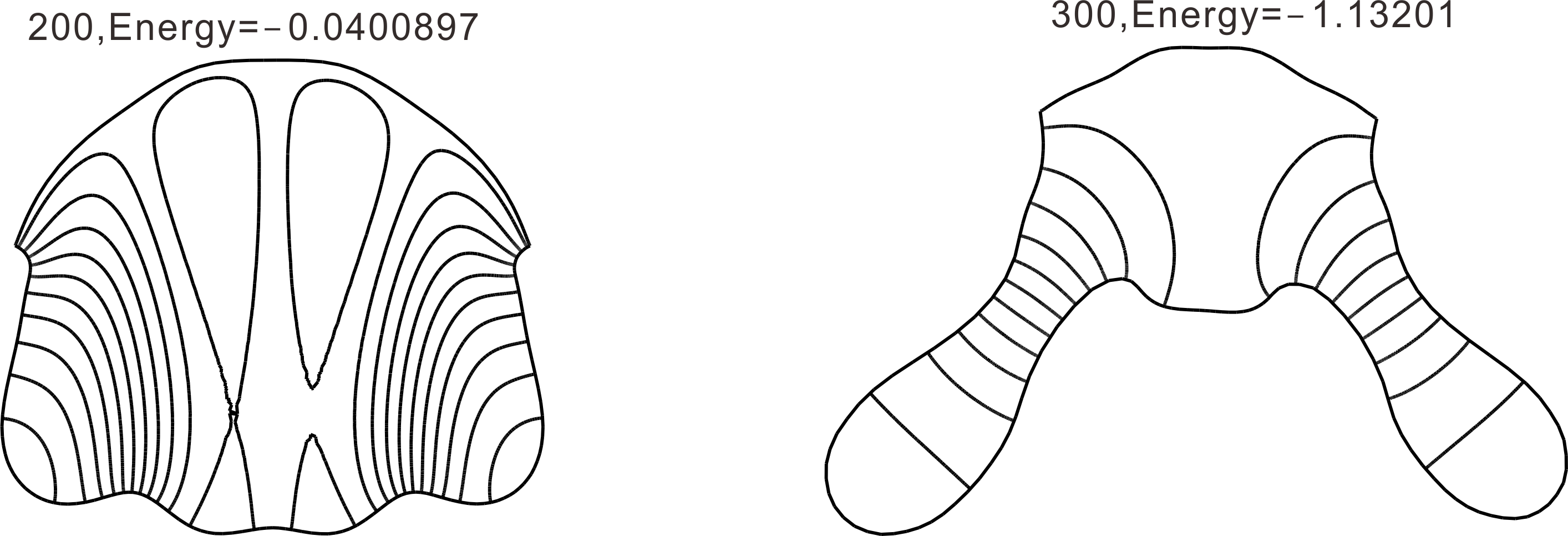A series of optimizations under $-\Delta u=\sin (\pi x_1)$.

## Mean Compliance Optimization

Cantilever by Allaire (see [Al07] 6.5 Algorithmes numériques)  More..
The initial domain $\Omega$ is just below. A series of domains $\Omega ^O_k$ satisfying the constraints $|\Omega ^O_k|=\textrm{constant}$ ($|D|$ represents the area of D.). $$F(\Omega )=\int _{\Gamma _N}g\cdot u\,ds$$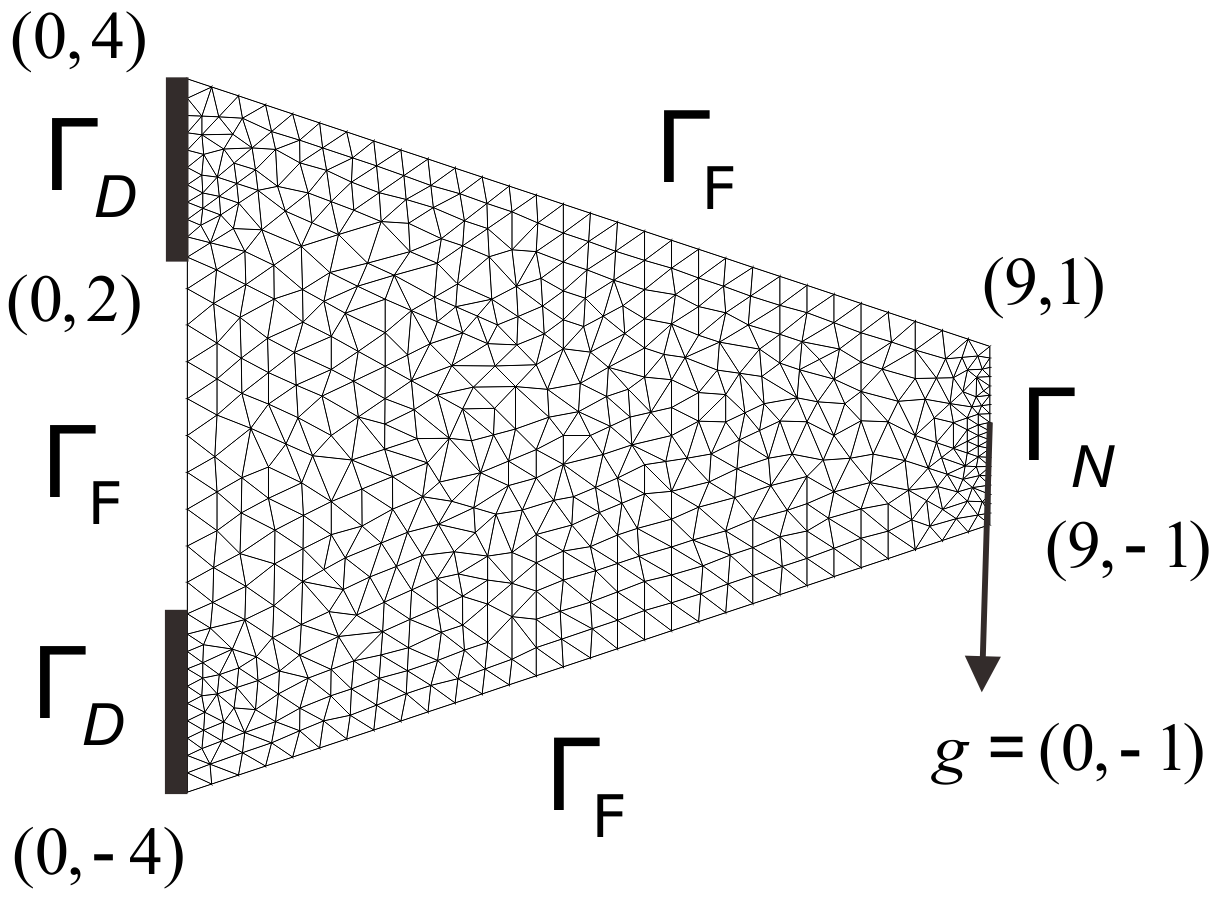where $\Gamma _N$ is fixed and vertical deformation of $\Gamma _D$ B is allowed. In [Al07], $\Gamma _D$ is fixed.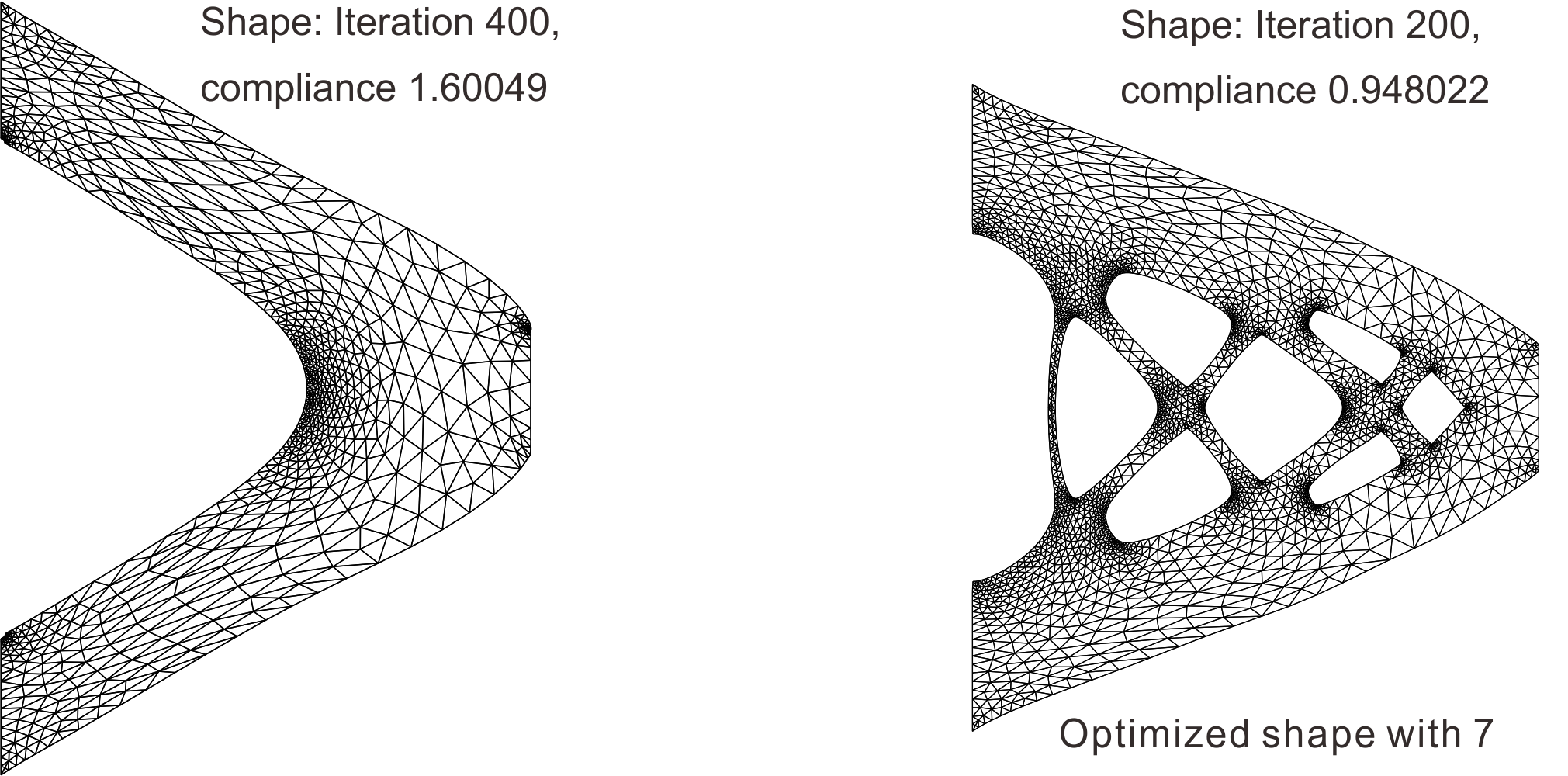The left side is the optimized shape in the figure just above, and the right side is the optimized shape with 7 holes.
Since the thinly stretched shape are more optimized, optimnal shape cannot be determined only by the constraint $|\Omega |$=constant.
More..

## Least Square Error

For a given functoin $u_d$, find $(u^O,\Omega ^O)$ such that $$F(u(\Omega )):=\int _{\Omega }|u(\Omega )-u_d|^2dx$$ The example below is an optimized shape when $u_d = 0$ in Poisson's equation under Dirichlet boundary conditions starting from the square region. Since it corresponds to the problem of cooling, it is considered that the longer the boundary, the more optimized domains.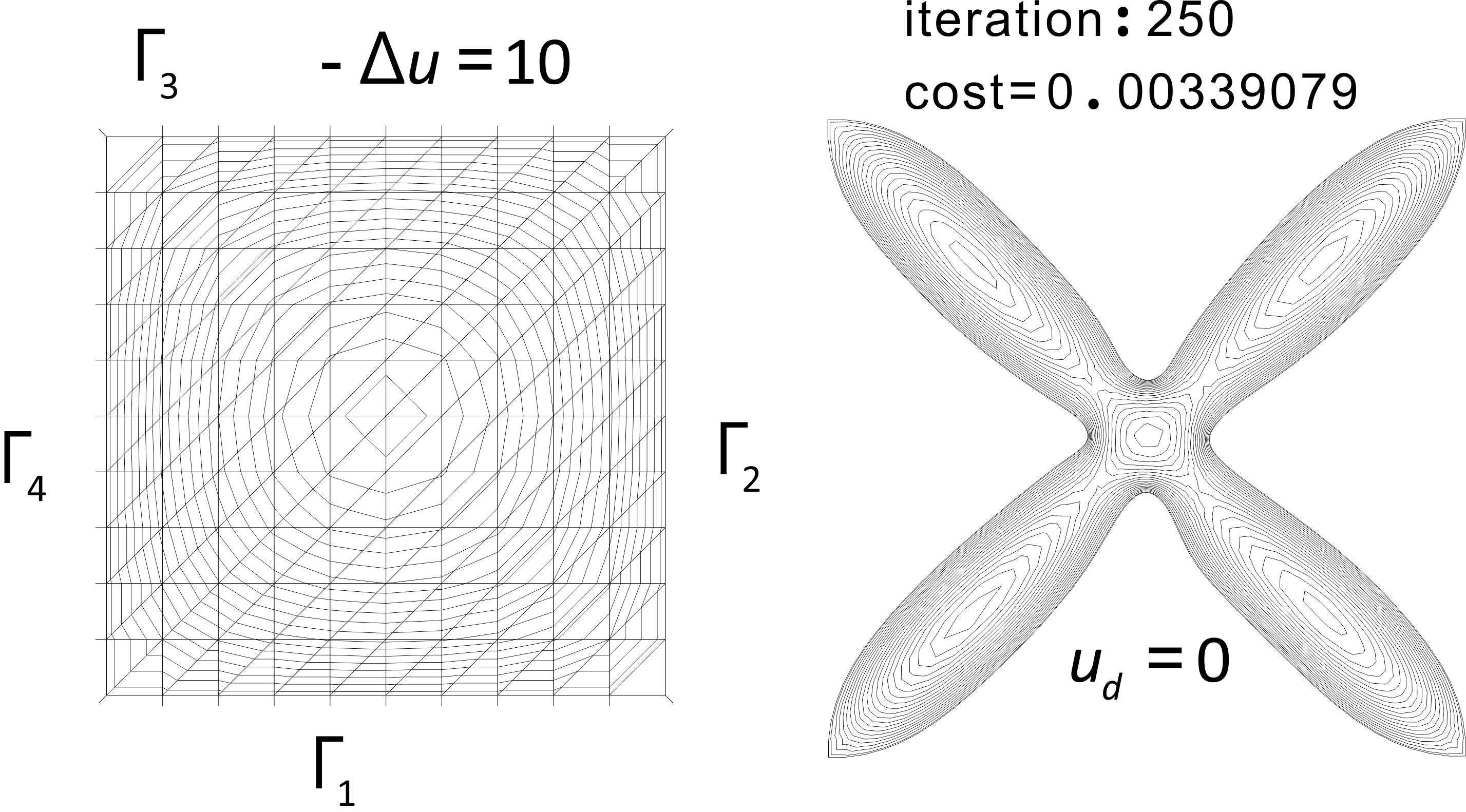The left side is the starting region, and an optimized domain in the right.
Next, consider the mixed boundary condition setting by $\Gamma _D=\Gamma _1,\Gamma _N=\Gamma _1\cup \Gamma _2\cup \Gamma _4$, and $u_d=0$. From the sequence below, it can be seen that $\Gamma _N^O$ approaches $\Gamma _D^O (u = 0)$ to reduce the error.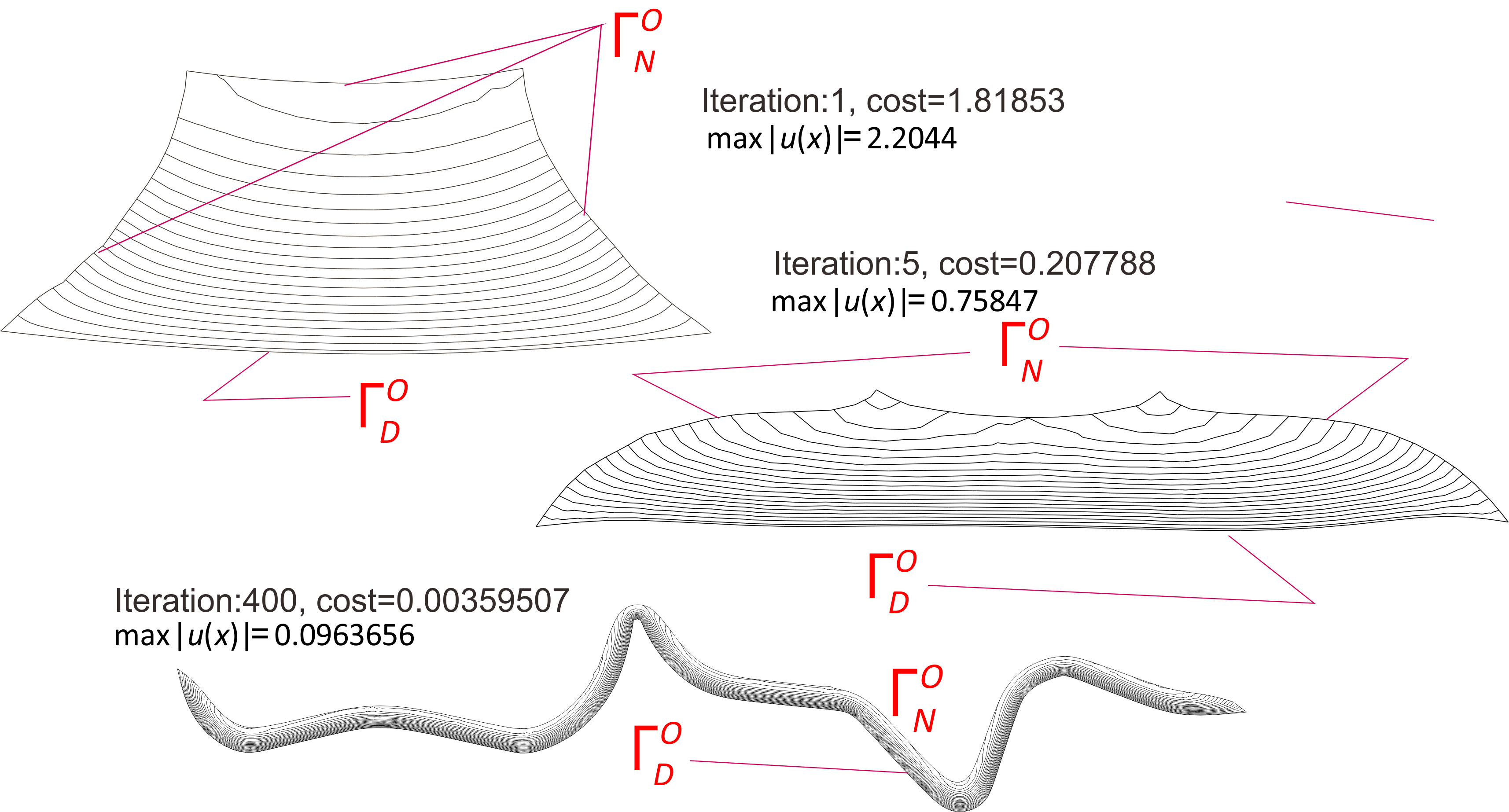At the 400th iteration, the optimized shape looks like an elongated string.
More..

## Shape optimization of Interfaces

Consider a composite in which two different elastic plates are joined together. The static shape is divided into $\Omega _1$ and $\Omega _2$ as shown on the left in the figure below, and Young's modulus are $E_1 = 200, E_2=20$ and Poisson's ratio are $\nu _1=0.25, \nu _2=0.3$. The initial compliance is $14.2113$ and the compliance after optimization is $12.092$ as shown in the figure on the right.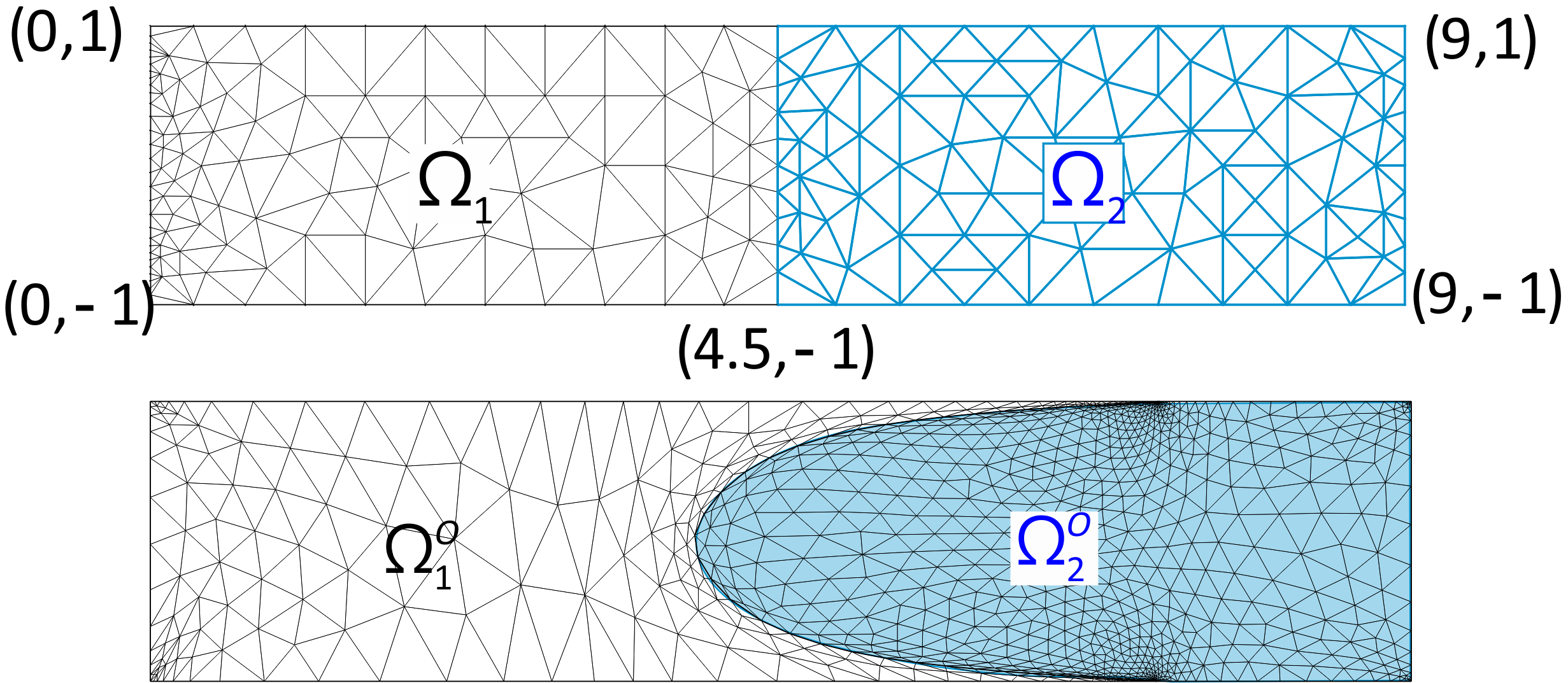More..

Information about the page: The current position is painted circle in the diagram below. Blue is the main MaKR and orange is a duplicate for MaKR's public use, where dashed line means the connection to the private area. The dashed lines are only connections to main MaKR.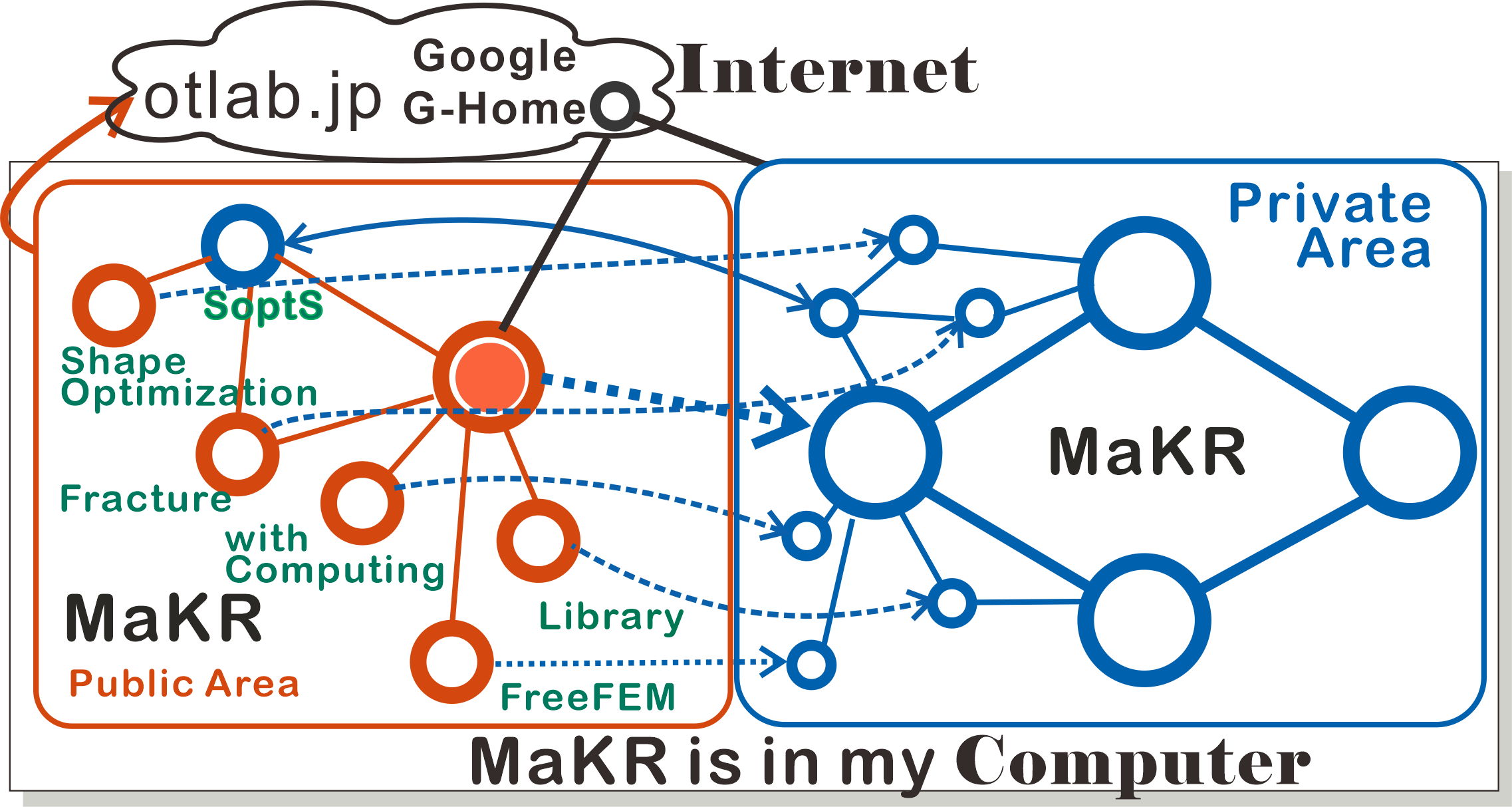## References

[A-P06] G. Allaire and O. Pantz, Structural optimization with FreeFem++, Struct. Multidiscip. Opt, 32 (2006), 173--181.
[Al07] G. Allaire, Conception optimale de structures, Springer, 2007.
[Az94] H. Azegami, Solution to domain optimization problems, Trans. Japan Soc. Mech. Engrs. Series A, 60, No.574 (1994), 1479--1486. (in Japanese)
[A-W96] H. Azegami and Z. Wu, Domain optimization analysis in linear elastic problems: Approach using traction method, JSME Inter. J. Series A, 39 (1996), 272--278.
[Az17] H. Azegami. Solution of shape optimization problem and its application to product design, Mathematical Analysis of Continuum Mechanics and Industrial Applications, Springer, 2017, 83--98.
[B-S04] M.P. Bends{\o }e and O. Sigmund, Topology optimization: theory, methods, and applications, Springer, 2004.
[Bu04] H.D. Bui, Fracture mechanics -- Inverse problems and solutions, Springer, 2006.
[Ch67] G.P. Cherepanov, On crack propagation in continuous media, Prikl. Math. Mekh., 31 (1967), 476--493.
[Cir88] P.G. Ciarlet, Mathematical elasticity: Three-dimensional elasticity, North-Holland, 1988.
[Co85] R. Correa and A. Seeger, Directional derivative of a minimax function. Nonlinear Anal., 9(1985), 13--22.
[D-Z88] M.C. Delfour and J.-P. Zolésio, Shape sensitivity analysis via min max differentiability, SIAM J. Control and Optim., 26(1988), 834--862.
[D-D81] Ph. Destuynder and M. Djaoua, Sur une interprétation de l'intégrale de Rice en théorie de la rupture fragile. Math. Meth. in Appl. Sci., 3 (1981), 70--87.
[E-G04] A. Em and J.-L. Guermond, Theory and practice of finite elements, Springer, 2004.
[Es56] J.D. Eshelby, The Continuum theory of lattice defects, Solid State Physics, 3 (1956), 79--144.
[F-O78] D. Fujiwara and S. Ozawa, The Hadamard variational formula for the Green functions of some normal elliptic boundary value problems, Proc. Japan Acad., 54 (1978), 215--220.
[G-S52] P.R. Garabedian and M. Schiffer, Convexity of domain functionals, J.Anal.Math., 2 (1952), 281--368.
[Gr21] A.A. Griffith, The phenomena of rupture and flow in solids, Phil. Trans. Roy. Soc. London, Series A 221 (1921), 163--198.
[Gr24] A.A. Griffith, The theory of rupture, Proc. 1st.Intern. Congr. Appl. Mech., Delft (1924) 55--63.
[Gr85] P. Grisvard, Elliptic problems in nonsmooth domains, Pitman, 1985.
[Gr92] P. Grisvard, Singularities in boundary value problems, Springer, 1992.
[Had68] J. Hadamard, Mémoire sur un problème d'analyse relatif à l'équilibre des plaques élastiques encastrées, Mémoire des savants étragers, 33 (1907), 515--629.
[Hau86] E.J. Haug, K.K. Choi and V. Komkov, Design sensitivity analysis of structural systems, Academic Press, 1986.
[ffempp] F. Hecht, New development in freefem++. J. Numer. Math. 20 (2012), 251--265. 65Y15, (FreeFem++ URL:http://www.freefem.org) %\url
[Kato] T. Kato, Perturbation theory for linear operators, Springer, 1980.
[K-W06] M. Kimura. and I. Wakano, New mathematical approach to the energy release rate in crack extension, Trans. Japan Soc. Indust. Appl. Math., 16(2006) 345--358. (in Japanese) \bibitem {K-W11} M. Kimura and I. Wakano, Shape derivative of potential energy and energy release rate in rracture mechanics, J. Math-for-industry, 3A (2011), 21--31.
[Kne05] D. Knees, Regularity results for quasilinear elliptic systems of power-law growth in nonsmooth domains: boundary, transmission and crack problems. PhD thesis, Universität Stuttgart, 2005. http://elib.uni-stuttgart.de/opus/volltexte/2005/2191/.
[Ko06] V.A. Kovtunenko, Primal-dual methods of shape sensitivity analysis for curvilinear cracks with nonpenetration, IMA Jour. Appl. Math. 71 (2006), 635--657.
[K-O18] V.A. Kovtunenko and K. Ohtsuka, Shape differentiability of Lagrangians and application to stokes problem, SIAM J. Control Optim. 56 (2018), 3668--3684.
[M-P01] B. Mohammadi and O. Pironneau, Applied shape optimization for fluids. Oxford University Press, 2001.
[Na94] S.Nazarov and B.A.Plamenevsky, Elliptic problems in domains with piecewise smooth boundaries, de Gruyter Expositions in Mathematics 13. Walter de Gruyter \& Co., 1994.
[Nec67] J. Necas, Direct methods in the theory of elliptic equations, Springer, 2012. Translated from Méthodes directes en théorie des équations elliptiques, 1967, Masson''.
[Noe18] E. Noether, Invariante variationsprobleme, göttinger nachrichten, Mathematisch-Physikalische Klasse (1918), 235--257.
[N-S13] A.A. Novotny and J. Sokolowski, Topological derivatives in shape optimization, Springer, 2013.
[Oh81] K. Ohtsuka, Generalized J-integral and three dimensional fracture mechanics I, Hiroshima Math. J., 11(1981), 21--52.
[Oh85] K. Ohtsuka, Generalized J-integral and its applications. I. -- Basic theory, Japan J. Appl. Math., 2 (1985), 329--350.
[O-K00] K. Ohtsuka and A. Khludnev, Generalized J-integral method for sensitivity analysis of static shape design, Control \& Cybernetics, 29 (2000), 513--533.
[Oh02] K. Ohtsuka, Comparison of criteria on the direction of crack extension, J. Comput. Appl. Math., 149 (2002), 335--339.
[Oh02-2] K. Ohtsuka, Theoretical and numerical analysis on 3-dimensional brittle fracture, Mathematical Modeling and Numerical Simulation in Continuum Mechanics, Springer, 2002, 233--251.
[Oh09] K. Ohtsuka, Criterion for stable/unstable quasi-static crack extension by extended griffith energy balance theory, Theor. Appl. Mech. Japan, 57 (2009), 25--32.
[Oh12] K. Ohtsuka, Shape optimization for partial differential equations/system with mixed boundary conditions, RIMS K\^oky\^uroku 1791 (2012), 172--181.
[OT-K12] K. Ohtsuka and M. Kimura, Differentiability of potential energies with a parameter and shape sensitivity analysis for nonlinear case: the p-Poisson problem, Japan J. Indust. Appl. Math., 29 (2012), 23--35.
[Oh14] K. Ohtsuka and T. Takaishi, Finite element anaysis using mathematical programming language FreeFem++, Kyoritsu Shuppan, 2014. (in Japanese)
[Oh17] K. Ohtsuka, Shape optimization by GJ-integral: Localization method for composite material, Mathematical Analysis of Continuum Mechanics and Industrial Applications, Springer, 2017, 73--109.
[Oh18] K. Ohtsuka, Shape optimization by Generalized J-integral in Poisson's equation with a mixed boundary condition, Mathematical Analysis of Continuum Mechanics and Industrial Applications II, Springer, 2018, 73--83.
[Pr10] A.N. Pressley, Elementary differential geometry, Springer, 2010.
[Ri68] J.R. Rice, A path-independent integral and the approximate analysis of strain concentration by notches and cracks, J. Appl. Mech., 35(1968), 379--386.
[Ri68-2] J.R. Rice, Mathematical analysis in the mechanics of fracture, Fracture Volume II, Academic Press, 1968, 191--311. %
[Pi84] O. Pironneau, Optimal shape design for elliptic systems, %Springer-Verlag, 1984.
[Sa99] J.A. Samareh, A survey of shape parameterization techniques, NASA Report CP-1999-209136 (1999), 333--343.
[Sc91] B.-W. Schulze, Pseudo-differential operators on manifolds with singularities, North-Holland, 1991.
[Sok92] J. Sokolowski and J.-P. Zolesio, Introduction to shape optimization, Springer, 1992.
[St14] K. Sturm, On shape optimization with non-linear partial differential equations, Doctoral thesis, Technische Universiltät of Berlin, 2014. https://d-nb.info/106856959X/34
[Sumi] Y. Sumi, Mathematical and computational analyses of cracking formation, Springer, 2014.
[Zei/2B] E. Zeidler. Nonlinear functional analysis and its applications II/B, Springer, 1990. %
[Z-S73] %O.C. Zienkiewicz and J.S. Campbell, Shape optimization and sequential %linear programming, Optimum Structural Design, Wiley, 1973, 109--126.
Top